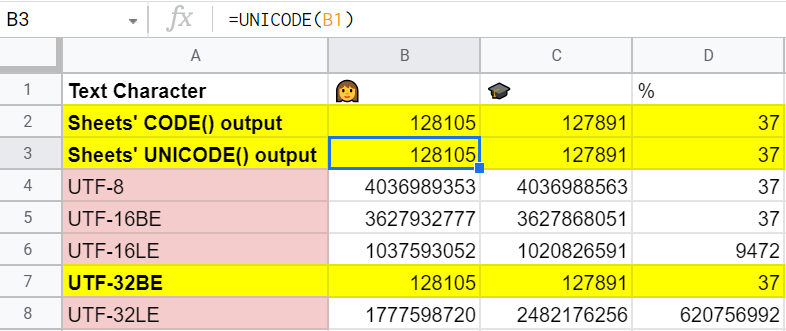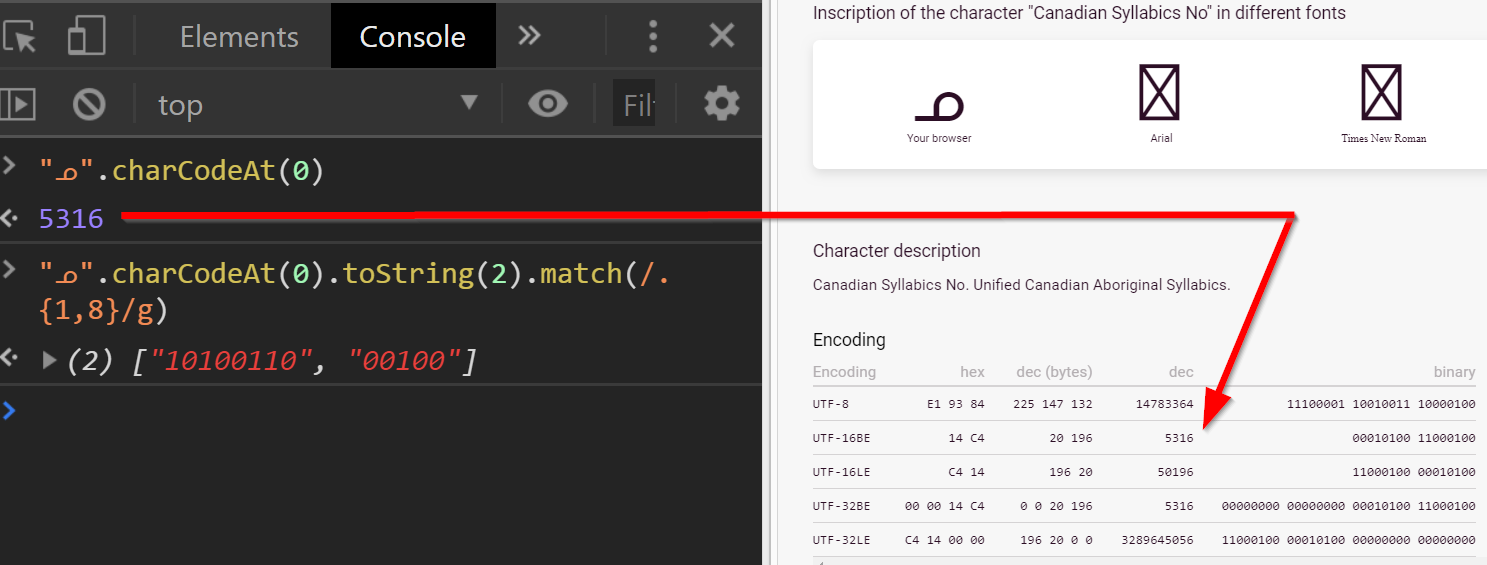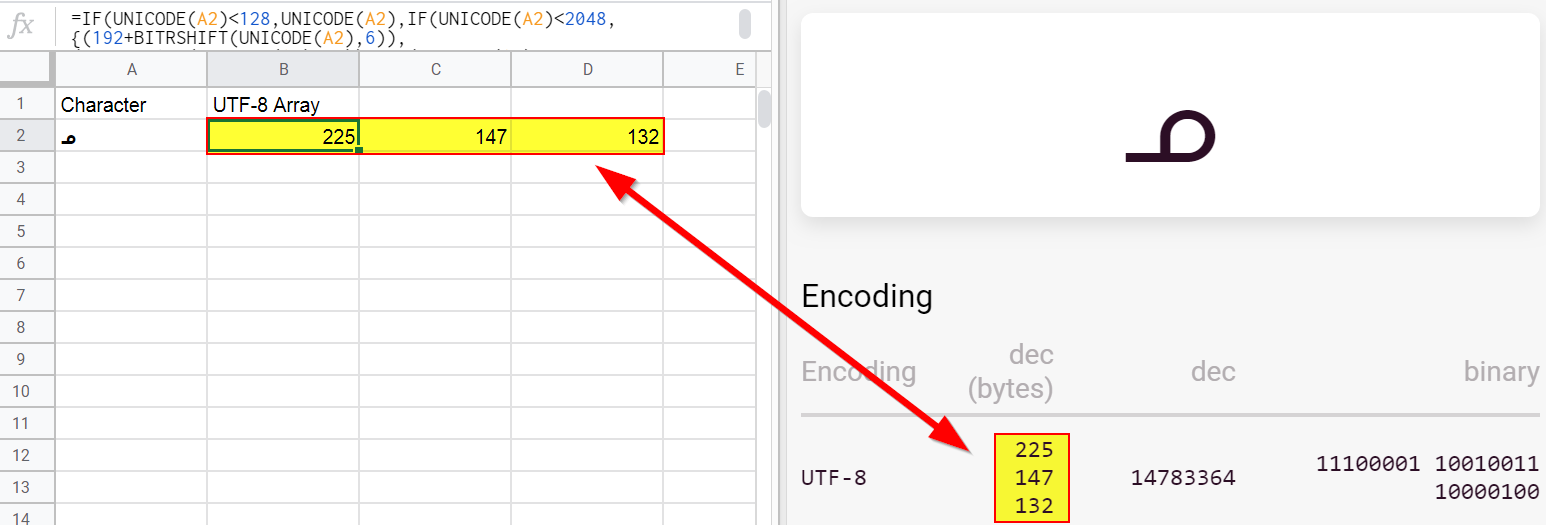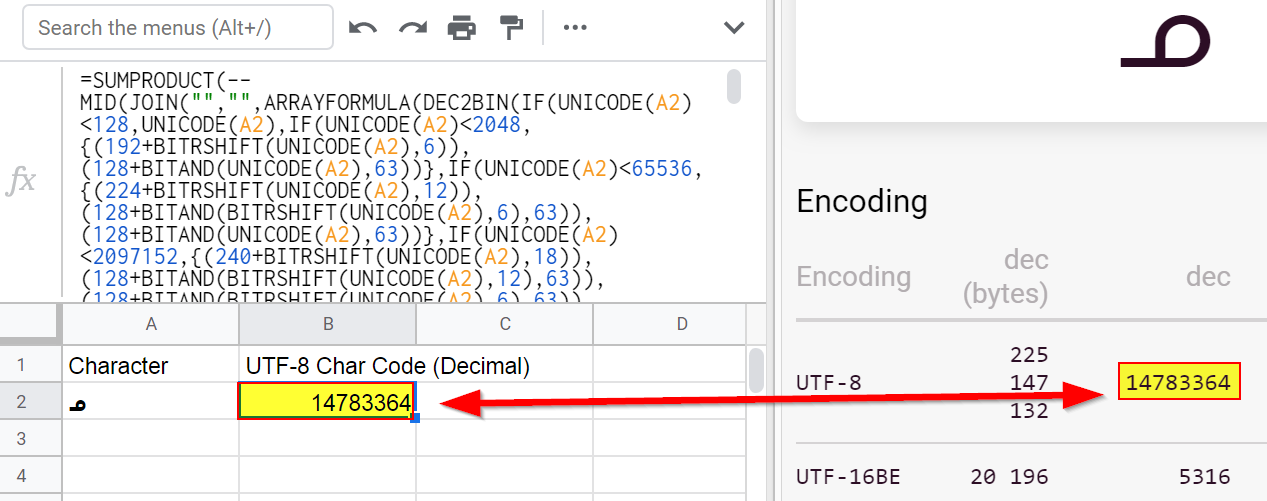# Google Sheets: UNICODE() Char Code to UTF-8, Decimal and Binary

• report
Disclaimer
Click for Disclaimer
This Post is over a year old (first published about 3 years ago). As such, please keep in mind that some of the information may no longer be accurate, best practice, or a reflection of how I would approach the same thing today.
• infoFull Post Details
info_outlineClick for Full Post Details
Date Posted:
Jun. 25, 2019
Last Updated:
Feb. 28, 2021
• classTags
classClick for Tags
Tags:

Warning: This post is going to get into the “dangerously nerdy” territory 🙂

## The issue – Google Sheets does not use UTF-8

I recently ran into a head-scratcher of an issue with Google Sheets, where a formula that used the output of the UNICODE() and/or CODE() functions was not matching with the expected value. After a bit of digging, I discovered that, although this is not really documented, most of the Google Sheets functions use UTF-32 (UTF-32BE) as the encoding scheme, whereas I was expecting UTF-8. This is made even more confusing by the fact that the Apps Script part of Google Sheets has an enum specifically for the UTF-8 charset.

To show this more clearly, here is table directly from Google Sheets, that show for various input characters, the `CODE()` function always returns the `UTF-32BE` value:Why is this a problem? Well, most of the time it is not; as long as you use the same encoding scheme on both ends (input and output) it really doesn’t matter. However, when you are feeding the result of UNICODE() into something where you don’t control the decoder part, it becomes an issue. To be specific, I was running into this with writing a custom Base64 encoder function (see full details on that here).

Usually, when people talk about converting text to Base64, without even saying it, the implication is that we are going to be using UTF-8 as the encoding scheme. In fact, the default charset used in Apps Script with Utilities.base64Decode() is UTF-8, and specifying UTF-32 for decoding is not an option! If you pull up various online “text to base64” converters online, chances are that 99% of them use either plain ASCII or UTF-8 for the encoding/decoding of text.

In my case, I was feeding the result of UNICODE() into my base64 generator, which was causing it to spit out a base64 encoded string that could only be properly decoded with a decoder that uses UTF-32, which most decoders do not use.

### Sidenote: JavaScript suffers the same issue!

This might surprise some people, but most JS engines do not use UTF-8 as the encoding scheme for strings – they use UTF-16!!! Similar to how I tested Google Sheets, this is easy to verify by checking the char code of a non-ASCII character:And guess where this gets special mention… the MDN page on base64! I am not alone in realizing the UTF-* issue with Base64!

## Why is UTF-8 so different?

The reason why UTF-8 is so different, and also why it is so popular, is because it is a variable length encoding scheme, with a minimum length of just one byte, and a maximum of 4 bytes wide. In comparison, UTF-16 is also variable, but has a fixed minimum of 2 bytes in width, and UTF-32 is not variable, and fixed at 4 bytes in width. The variable nature of UTF-8 means that each byte also has to have information in it on whether or not it is the last byte, which is the reason why you can’t just plug the binary from a 2-byte-wide UTF-8 char into UTF-32, and vice-versa.

## The solutions!

There is a lot to this, so I’m going to break down my overall solution (Text -> UTF-16 Unicode Decimal -> UTF-8 Decimal -> UTF-8 Binary) into smaller chunks, in case you need just one part of it.

### Google Sheets: Text Character to UTF-8 Array

This section talks about converting UTF-16 to UTF-8. Originally, I thought Sheets was using UTf-16, but I am now aware it is UTF-32BE. However, the approach below still seems to work.

My starting point for this project was to try and convert a single UTF-16 charcode/codepoint to a UTF-8 codepoint. Luckily, the MDN page on base64 encoding and decoding had a very clean Javascript function I could use as a base.

Here is the relevant part of their Javascript code, that converts a UTF8 charcode to UTF-16:

``````nChr = sDOMStr.charCodeAt(nChrIdx);
if (nChr < 128) {
/* one byte */
aBytes[nIdx++] = nChr;
} else if (nChr < 0x800) {
/* two bytes */
aBytes[nIdx++] = 192 + (nChr >>> 6);
aBytes[nIdx++] = 128 + (nChr & 63);
} else if (nChr < 0x10000) {
/* three bytes */
aBytes[nIdx++] = 224 + (nChr >>> 12);
aBytes[nIdx++] = 128 + (nChr >>> 6 & 63);
aBytes[nIdx++] = 128 + (nChr & 63);
} else if (nChr < 0x200000) {
/* four bytes */
aBytes[nIdx++] = 240 + (nChr >>> 18);
aBytes[nIdx++] = 128 + (nChr >>> 12 & 63);
aBytes[nIdx++] = 128 + (nChr >>> 6 & 63);
aBytes[nIdx++] = 128 + (nChr & 63);
} else if (nChr < 0x4000000) {
/* five bytes */
aBytes[nIdx++] = 248 + (nChr >>> 24);
aBytes[nIdx++] = 128 + (nChr >>> 18 & 63);
aBytes[nIdx++] = 128 + (nChr >>> 12 & 63);
aBytes[nIdx++] = 128 + (nChr >>> 6 & 63);
aBytes[nIdx++] = 128 + (nChr & 63);
} else /* if (nChr <= 0x7fffffff) */ {
/* six bytes */
aBytes[nIdx++] = 252 + (nChr >>> 30);
aBytes[nIdx++] = 128 + (nChr >>> 24 & 63);
aBytes[nIdx++] = 128 + (nChr >>> 18 & 63);
aBytes[nIdx++] = 128 + (nChr >>> 12 & 63);
aBytes[nIdx++] = 128 + (nChr >>> 6 & 63);
aBytes[nIdx++] = 128 + (nChr & 63);
}
``````

And, here it is replicated in Google Sheets, as a formula:

``````=IF(UNICODE(A2)<128,UNICODE(A2),IF(UNICODE(A2)<2048,{(192+BITRSHIFT(UNICODE(A2),6)),(128+BITAND(UNICODE(A2),63))},IF(UNICODE(A2)<65536,{(224+BITRSHIFT(UNICODE(A2),12)),(128+BITAND(BITRSHIFT(UNICODE(A2),6),63)),(128+BITAND(UNICODE(A2),63))},IF(UNICODE(A2)<2097152,{(240+BITRSHIFT(UNICODE(A2),18)),(128+BITAND(BITRSHIFT(UNICODE(A2),12),63)),(128+BITAND(BITRSHIFT(UNICODE(A2),6),63)),(128+BITAND(UNICODE(A2),63))},IF(UNICODE(A2)<67108864,{(248+BITRSHIFT(UNICODE(A2),24)),(128+BITAND(BITRSHIFT(UNICODE(A2),18),63)),(128+BITAND(BITRSHIFT(UNICODE(A2),12),63)),(128+BITAND(BITRSHIFT(UNICODE(A2),6),63)),(128+BITAND(UNICODE(A2),63))},{(248+BITRSHIFT(UNICODE(A2),30)),(128+BITAND(BITRSHIFT(UNICODE(A2),24),63)),(128+BITAND(BITRSHIFT(UNICODE(A2),18),63)),(128+BITAND(BITRSHIFT(UNICODE(A2),12),63)),(128+BITAND(BITRSHIFT(UNICODE(A2),6),63)),(128+BITAND(UNICODE(A2),63))})))))
``````

Or, if Google Sheets doesn’t like the nested IFS returning arrays of different sizes, this slightly longer formula uses SPLIT() to produce the arrays:

``````=IF(UNICODE(A2)<128,UNICODE(A2),IF(UNICODE(A2)<2048,SPLIT(JOIN("|",(192+BITRSHIFT(UNICODE(A2),6)),(128+BITAND(UNICODE(A2),63))),"|"),IF(UNICODE(A2)<65536,SPLIT(JOIN("|",(224+BITRSHIFT(UNICODE(A2),12)),(128+BITAND(BITRSHIFT(UNICODE(A2),6),63)),(128+BITAND(UNICODE(A2),63))),"|"),IF(UNICODE(A2)<2097152,SPLIT(JOIN("|",(240+BITRSHIFT(UNICODE(A2),18)),(128+BITAND(BITRSHIFT(UNICODE(A2),12),63)),(128+BITAND(BITRSHIFT(UNICODE(A2),6),63)),(128+BITAND(UNICODE(A2),63))),"|"),IF(UNICODE(A2)<67108864,SPLIT(JOIN("|",(248+BITRSHIFT(UNICODE(A2),24)),(128+BITAND(BITRSHIFT(UNICODE(A2),18),63)),(128+BITAND(BITRSHIFT(UNICODE(A2),12),63)),(128+BITAND(BITRSHIFT(UNICODE(A2),6),63)),(128+BITAND(UNICODE(A2),63))),"|"),SPLIT(JOIN("|",(248+BITRSHIFT(UNICODE(A2),30)),(128+BITAND(BITRSHIFT(UNICODE(A2),24),63)),(128+BITAND(BITRSHIFT(UNICODE(A2),18),63)),(128+BITAND(BITRSHIFT(UNICODE(A2),12),63)),(128+BITAND(BITRSHIFT(UNICODE(A2),6),63)),(128+BITAND(UNICODE(A2),63))),"|"))))))
``````

And here is confirmation that it works:### Google Sheets: Text to UTF-8 Binary

The formula above is cool, but it spits out the code points as an array, chunked in bytes, represented in decimal. What if we want it in binary? And how about as a single cell instead of as an array? That would be neat!

OK! Let’s do it! First, lets convert the decimal array into binary. To do that, the easiest way will be to apply the DEC2BIN() function to each element of the array, converting from decimal to binary, which can be done by wrapping the above formula in =ARRAYFORMULA(DEC2BIN(…)). Easy!

And if we want it as a single cell output instead of an array? Just use JOIN()! Here is the final massive formula, to convert a text cell into UTF-8 binary:

``````=JOIN("","",ARRAYFORMULA(DEC2BIN(IF(UNICODE(A2)<128,UNICODE(A2),IF(UNICODE(A2)<2048,SPLIT(JOIN("|",(192+BITRSHIFT(UNICODE(A2),6)),(128+BITAND(UNICODE(A2),63))),"|"),IF(UNICODE(A2)<65536,SPLIT(JOIN("|",(224+BITRSHIFT(UNICODE(A2),12)),(128+BITAND(BITRSHIFT(UNICODE(A2),6),63)),(128+BITAND(UNICODE(A2),63))),"|"),IF(UNICODE(A2)<2097152,SPLIT(JOIN("|",(240+BITRSHIFT(UNICODE(A2),18)),(128+BITAND(BITRSHIFT(UNICODE(A2),12),63)),(128+BITAND(BITRSHIFT(UNICODE(A2),6),63)),(128+BITAND(UNICODE(A2),63))),"|"),IF(UNICODE(A2)<67108864,SPLIT(JOIN("|",(248+BITRSHIFT(UNICODE(A2),24)),(128+BITAND(BITRSHIFT(UNICODE(A2),18),63)),(128+BITAND(BITRSHIFT(UNICODE(A2),12),63)),(128+BITAND(BITRSHIFT(UNICODE(A2),6),63)),(128+BITAND(UNICODE(A2),63))),"|"),SPLIT(JOIN("|",(248+BITRSHIFT(UNICODE(A2),30)),(128+BITAND(BITRSHIFT(UNICODE(A2),24),63)),(128+BITAND(BITRSHIFT(UNICODE(A2),18),63)),(128+BITAND(BITRSHIFT(UNICODE(A2),12),63)),(128+BITAND(BITRSHIFT(UNICODE(A2),6),63)),(128+BITAND(UNICODE(A2),63))),"|")))))))))
``````

### Google Sheets: Text to UTF-8 Decimal (Pure Formula)

Based on what we have so far, we should just be able to shove the final large binary block generated by the above formula in to BIN2DEC(), right?

Error: Function BIN2DEC parameter 1 is too long. It is 24 characters; the maximum length is 10 characters.

Uh-oh! Well, that is disappointing. But here is an alternative; instead of using “character -> utf-16 -> utf-8 split into bytes as decimal -> split into bytes as binary -> binary”, we can stop at the 3rd step, where we have an array of bytes as decimals, and add them together into a binary product. I have to admit that binary math is a bit beyond the scope of what I normally am comfortable with, but I managed to hack this together with a related answer I found for working around the BIN2DEC limit with Excel. Here is the final formula!

``````=SUMPRODUCT(--MID(JOIN("","",ARRAYFORMULA(DEC2BIN(IF(UNICODE(A2)<128,UNICODE(A2),IF(UNICODE(A2)<2048,{(192+BITRSHIFT(UNICODE(A2),6)),(128+BITAND(UNICODE(A2),63))},IF(UNICODE(A2)<65536,{(224+BITRSHIFT(UNICODE(A2),12)),(128+BITAND(BITRSHIFT(UNICODE(A2),6),63)),(128+BITAND(UNICODE(A2),63))},IF(UNICODE(A2)<2097152,{(240+BITRSHIFT(UNICODE(A2),18)),(128+BITAND(BITRSHIFT(UNICODE(A2),12),63)),(128+BITAND(BITRSHIFT(UNICODE(A2),6),63)),(128+BITAND(UNICODE(A2),63))},IF(UNICODE(A2)<67108864,{(248+BITRSHIFT(UNICODE(A2),24)),(128+BITAND(BITRSHIFT(UNICODE(A2),18),63)),(128+BITAND(BITRSHIFT(UNICODE(A2),12),63)),(128+BITAND(BITRSHIFT(UNICODE(A2),6),63)),(128+BITAND(UNICODE(A2),63))},{(248+BITRSHIFT(UNICODE(A2),30)),(128+BITAND(BITRSHIFT(UNICODE(A2),24),63)),(128+BITAND(BITRSHIFT(UNICODE(A2),18),63)),(128+BITAND(BITRSHIFT(UNICODE(A2),12),63)),(128+BITAND(BITRSHIFT(UNICODE(A2),6),63)),(128+BITAND(UNICODE(A2),63))})))))))),LEN(JOIN("","",ARRAYFORMULA(DEC2BIN(IF(UNICODE(A2)<128,UNICODE(A2),IF(UNICODE(A2)<2048,{(192+BITRSHIFT(UNICODE(A2),6)),(128+BITAND(UNICODE(A2),63))},IF(UNICODE(A2)<65536,{(224+BITRSHIFT(UNICODE(A2),12)),(128+BITAND(BITRSHIFT(UNICODE(A2),6),63)),(128+BITAND(UNICODE(A2),63))},IF(UNICODE(A2)<2097152,{(240+BITRSHIFT(UNICODE(A2),18)),(128+BITAND(BITRSHIFT(UNICODE(A2),12),63)),(128+BITAND(BITRSHIFT(UNICODE(A2),6),63)),(128+BITAND(UNICODE(A2),63))},IF(UNICODE(A2)<67108864,{(248+BITRSHIFT(UNICODE(A2),24)),(128+BITAND(BITRSHIFT(UNICODE(A2),18),63)),(128+BITAND(BITRSHIFT(UNICODE(A2),12),63)),(128+BITAND(BITRSHIFT(UNICODE(A2),6),63)),(128+BITAND(UNICODE(A2),63))},{(248+BITRSHIFT(UNICODE(A2),30)),(128+BITAND(BITRSHIFT(UNICODE(A2),24),63)),(128+BITAND(BITRSHIFT(UNICODE(A2),18),63)),(128+BITAND(BITRSHIFT(UNICODE(A2),12),63)),(128+BITAND(BITRSHIFT(UNICODE(A2),6),63)),(128+BITAND(UNICODE(A2),63))})))))))))+1-ROW(INDIRECT("1:"&LEN(JOIN("","",ARRAYFORMULA(DEC2BIN(IF(UNICODE(A2)<128,UNICODE(A2),IF(UNICODE(A2)<2048,{(192+BITRSHIFT(UNICODE(A2),6)),(128+BITAND(UNICODE(A2),63))},IF(UNICODE(A2)<65536,{(224+BITRSHIFT(UNICODE(A2),12)),(128+BITAND(BITRSHIFT(UNICODE(A2),6),63)),(128+BITAND(UNICODE(A2),63))},IF(UNICODE(A2)<2097152,{(240+BITRSHIFT(UNICODE(A2),18)),(128+BITAND(BITRSHIFT(UNICODE(A2),12),63)),(128+BITAND(BITRSHIFT(UNICODE(A2),6),63)),(128+BITAND(UNICODE(A2),63))},IF(UNICODE(A2)<67108864,{(248+BITRSHIFT(UNICODE(A2),24)),(128+BITAND(BITRSHIFT(UNICODE(A2),18),63)),(128+BITAND(BITRSHIFT(UNICODE(A2),12),63)),(128+BITAND(BITRSHIFT(UNICODE(A2),6),63)),(128+BITAND(UNICODE(A2),63))},{(248+BITRSHIFT(UNICODE(A2),30)),(128+BITAND(BITRSHIFT(UNICODE(A2),24),63)),(128+BITAND(BITRSHIFT(UNICODE(A2),18),63)),(128+BITAND(BITRSHIFT(UNICODE(A2),12),63)),(128+BITAND(BITRSHIFT(UNICODE(A2),6),63)),(128+BITAND(UNICODE(A2),63))}))))))))))),1),(2^(ROW(INDIRECT("1:"&LEN(JOIN("","",ARRAYFORMULA(DEC2BIN(IF(UNICODE(A2)<128,UNICODE(A2),IF(UNICODE(A2)<2048,{(192+BITRSHIFT(UNICODE(A2),6)),(128+BITAND(UNICODE(A2),63))},IF(UNICODE(A2)<65536,{(224+BITRSHIFT(UNICODE(A2),12)),(128+BITAND(BITRSHIFT(UNICODE(A2),6),63)),(128+BITAND(UNICODE(A2),63))},IF(UNICODE(A2)<2097152,{(240+BITRSHIFT(UNICODE(A2),18)),(128+BITAND(BITRSHIFT(UNICODE(A2),12),63)),(128+BITAND(BITRSHIFT(UNICODE(A2),6),63)),(128+BITAND(UNICODE(A2),63))},IF(UNICODE(A2)<67108864,{(248+BITRSHIFT(UNICODE(A2),24)),(128+BITAND(BITRSHIFT(UNICODE(A2),18),63)),(128+BITAND(BITRSHIFT(UNICODE(A2),12),63)),(128+BITAND(BITRSHIFT(UNICODE(A2),6),63)),(128+BITAND(UNICODE(A2),63))},{(248+BITRSHIFT(UNICODE(A2),30)),(128+BITAND(BITRSHIFT(UNICODE(A2),24),63)),(128+BITAND(BITRSHIFT(UNICODE(A2),18),63)),(128+BITAND(BITRSHIFT(UNICODE(A2),12),63)),(128+BITAND(BITRSHIFT(UNICODE(A2),6),63)),(128+BITAND(UNICODE(A2),63))})))))))))))-1)))
``````

And here is the confirmation it works:Pretty neat, huh?

### Google Sheets: Text to UTF-8 Decimal (Javascript)

As a “cleaner” solution, that does not use a huge formula, we can take advantage of Google Sheets custom formulas, which we can setup with Javascript in “Tools -> Script editor”. Here is the code to use:

``````function utf8Char(input) {
var decArr = strToUTF8Arr(input);
var binString = '';
for (var x = 0; x < decArr.length; x++) {
binString += (decArr[x]).toString(2);
}
return parseInt(binString, 2);
}

function strToUTF8Arr(input) {
var aBytes, nChr, nStrLen = input.length, nArrLen = 0;
for (var nMapIdx = 0; nMapIdx < nStrLen; nMapIdx++) {
nChr = input.charCodeAt(nMapIdx);
nArrLen += nChr < 0x80 ? 1 : nChr < 0x800 ? 2 : nChr < 0x10000 ? 3 : nChr < 0x200000 ? 4 : nChr < 0x4000000 ? 5 : 6;
}
aBytes = [];
for (var x = 0; x < nArrLen.length; x++) {
aBytes.push(nArrLen[x] < 0 ? nArrLen[x] + 256 : nArrLen[x]);
}
for (var nIdx = 0, nChrIdx = 0; nIdx < nArrLen; nChrIdx++) {
nChr = input.charCodeAt(nChrIdx);
if (nChr < 128) {
/* one byte */
aBytes[nIdx++] = nChr;
} else if (nChr < 0x800) {
/* two bytes */
aBytes[nIdx++] = 192 + (nChr >>> 6);
aBytes[nIdx++] = 128 + (nChr & 63);
} else if (nChr < 0x10000) {
/* three bytes */
aBytes[nIdx++] = 224 + (nChr >>> 12);
aBytes[nIdx++] = 128 + (nChr >>> 6 & 63);
aBytes[nIdx++] = 128 + (nChr & 63);
} else if (nChr < 0x200000) {
/* four bytes */
aBytes[nIdx++] = 240 + (nChr >>> 18);
aBytes[nIdx++] = 128 + (nChr >>> 12 & 63);
aBytes[nIdx++] = 128 + (nChr >>> 6 & 63);
aBytes[nIdx++] = 128 + (nChr & 63);
} else if (nChr < 0x4000000) {
/* five bytes */
aBytes[nIdx++] = 248 + (nChr >>> 24);
aBytes[nIdx++] = 128 + (nChr >>> 18 & 63);
aBytes[nIdx++] = 128 + (nChr >>> 12 & 63);
aBytes[nIdx++] = 128 + (nChr >>> 6 & 63);
aBytes[nIdx++] = 128 + (nChr & 63);
} else /* if (nChr <= 0x7fffffff) */ {
/* six bytes */
aBytes[nIdx++] = 252 + (nChr >>> 30);
aBytes[nIdx++] = 128 + (nChr >>> 24 & 63);
aBytes[nIdx++] = 128 + (nChr >>> 18 & 63);
aBytes[nIdx++] = 128 + (nChr >>> 12 & 63);
aBytes[nIdx++] = 128 + (nChr >>> 6 & 63);
aBytes[nIdx++] = 128 + (nChr & 63);
}
}
return aBytes;
}
``````

Simply copy and paste this code into the script editor, save, and reload your spreadsheet. After that, you can use:

`=utf8Char(A2)`

…and get back the UTF-8 charcode in decimal!

2/28/2021 Update: I previously thought that Sheets was using `UTF-16`, but after a revisit to this post, I realized it is actually `UTF-32BE`; the post has been updated to reflect this.

## 2 thoughts on “Google Sheets: UNICODE() Char Code to UTF-8, Decimal and Binary”

1.Adel says:

Google Sheets – Text to UTF-8 Char Code

this link not work! ( MSG : Oops! That page can’t be found. )

1.joshuatz says:

I’m going to assume this was about one of the “proof” screenshots – fixed now, just needed to be uploaded! Thanks for pointing this out!